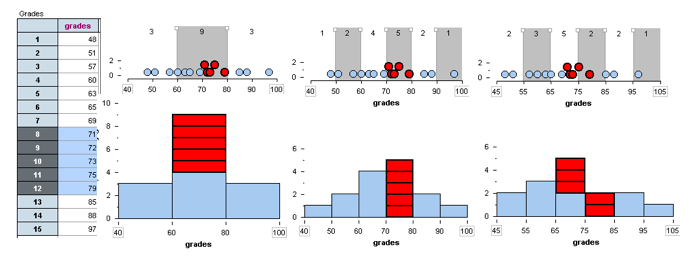## Histograms (2 of 4)

### Learning Objectives

• Describe the distribution of quantitative data using a histogram.

We have discussed two types of graphs that summarize a distribution of a quantitative variable: dotplots and histograms.

From a dotplot, we also described the pattern in the data with statements about shape, center, and spread. We have to be more cautious making similar statements using a histogram because our perception of shape, center, and spread can be affected by how the bins are defined. We investigate this important point in the next example.

### Example

We used the same set of data to construct these three histograms of student scores. Are you surprised by how different the distribution looks in each histogram?The histogram on the left has a bin width of 20. The first bin starts at 40. To create the middle histogram, we changed the bin width to 10 but kept the first bin starting at 40. To create the last histogram, we kept the bin width at 10 but started the first bin at 45.

These changes affect our description of the shape, center, and spread of this set of data. For example, in the histogram on the left, the distribution looks symmetric with a central peak. In the histogram on the right, the distribution looks slightly skewed to the right. Based on the middle histogram, we might estimate that most students scored between 70 and 80. But the histogram on the right suggests that typical students scored between 65 and 75.

Why does changing the bin size and the starting point of the first bin change the histogram so drastically?

When we change the bins, the data gets grouped differently. The different grouping affects the appearance of the histogram.

To illustrate this point, we highlighted the five students who scored in the 70s in each histogram.

• In the histogram on the left, these five students are grouped in the middle bin with other students who scored between 60 and 80.
• In the histogram in the middle, these five students form a bin of their own, since no other students scored between 70 and 80.
• In the histogram on the right, these five students are in separate bins.Which histogram gives the most helpful summary of the distribution?

For this situation, the middle histogram is probably the most useful summary because the intervals correspond to letter grades.

Our general advice is as follows:

• Avoid histograms with large bin widths that group data into only a few bins. A histogram constructed with large bin widths will show the distribution as a “skyscraper.” This does not give good information about variability in the distribution.
• Avoid histograms with small bin widths that group data into lots of bins. A histogram constructed with small bin widths will show the distribution as a “pancake.” This does not help us see the pattern in the data.

Use the simulation below to answer the questions in the next Learn By Doing.